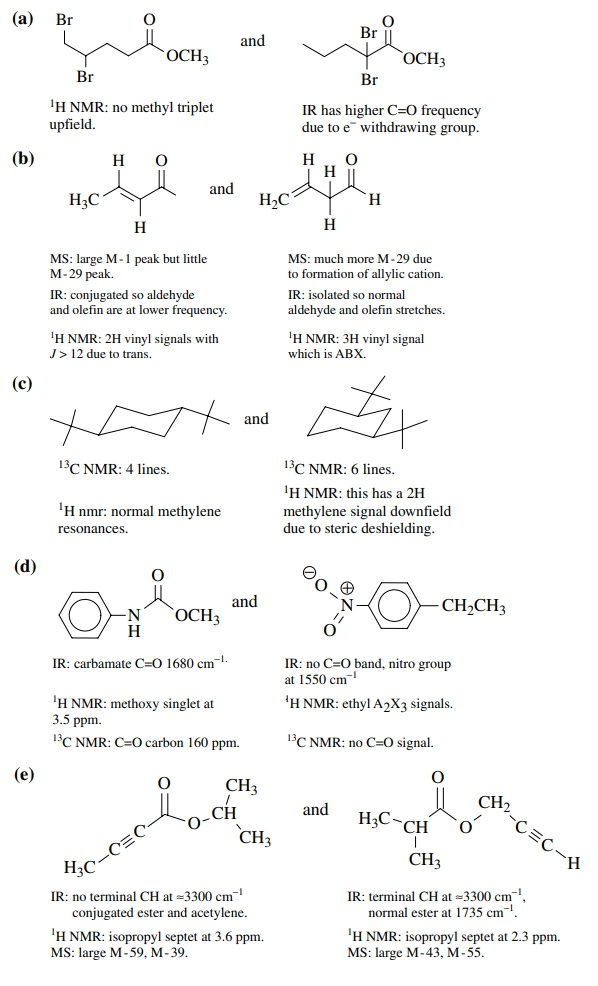# Solved Problems on Structure Determination of Organic Compounds

| Home | | Organic Chemistry |

## Chapter: Organic Chemistry : Structure Determination of Organic Compounds

Solved Problems on Structure Determination of Organic Compounds - Organic Chemistry

PROBLEMS

11.1. For the following compounds, label the spin systems present, label the symmetry properties of protons where possible (homotopic, enantiotopic, diastereotopic), and predict the splitting pattern for the proton(s) indicated by an arrow.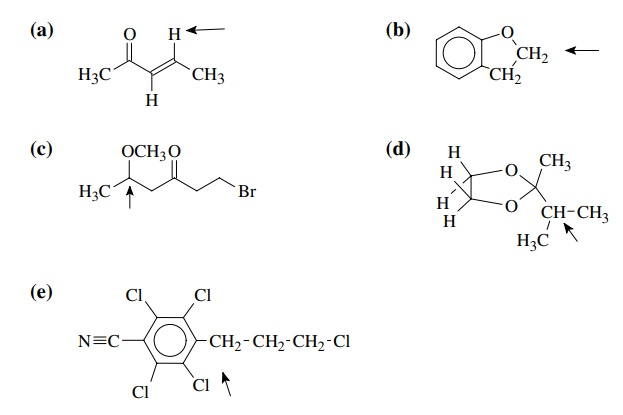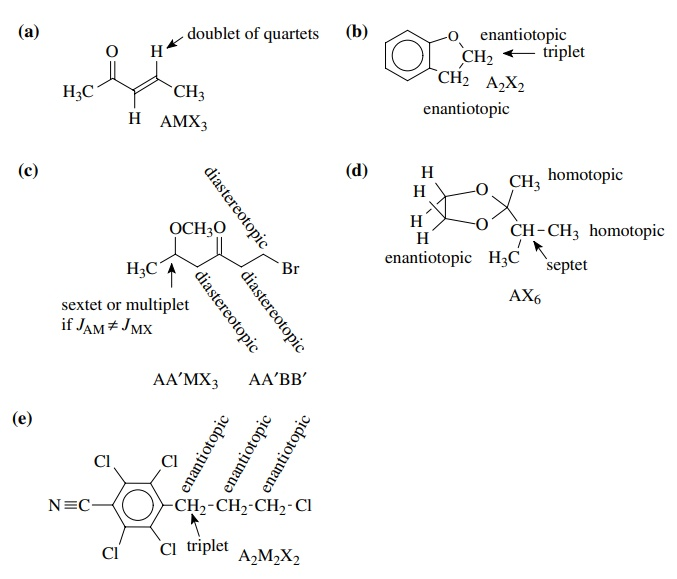11.2. Tell how you could use 1H NMR to distinguish the following pairs of compounds. Be specific as to what data you would look for and how you would interpret it. There might be more than one feature in the 1H NMR that could be used so give a complete answer.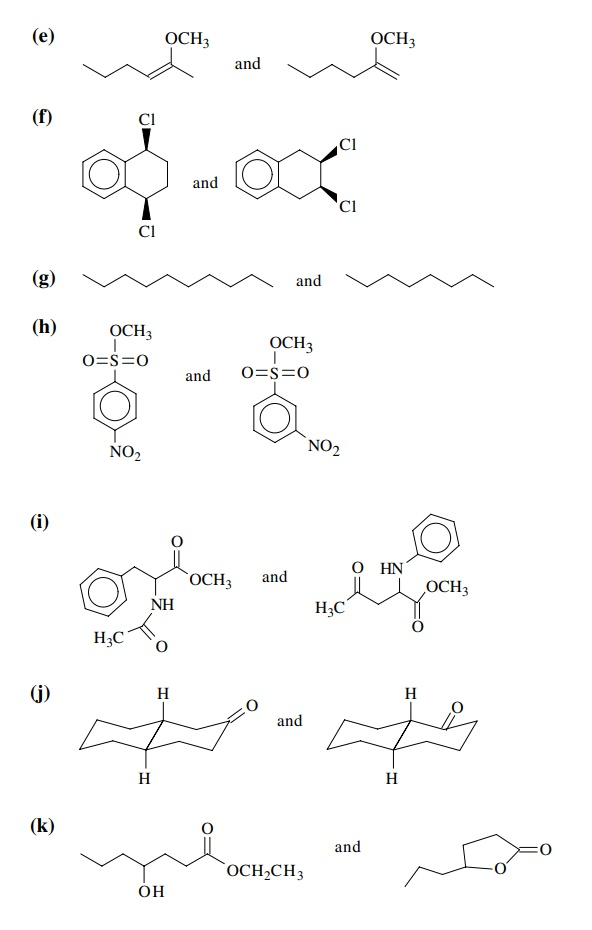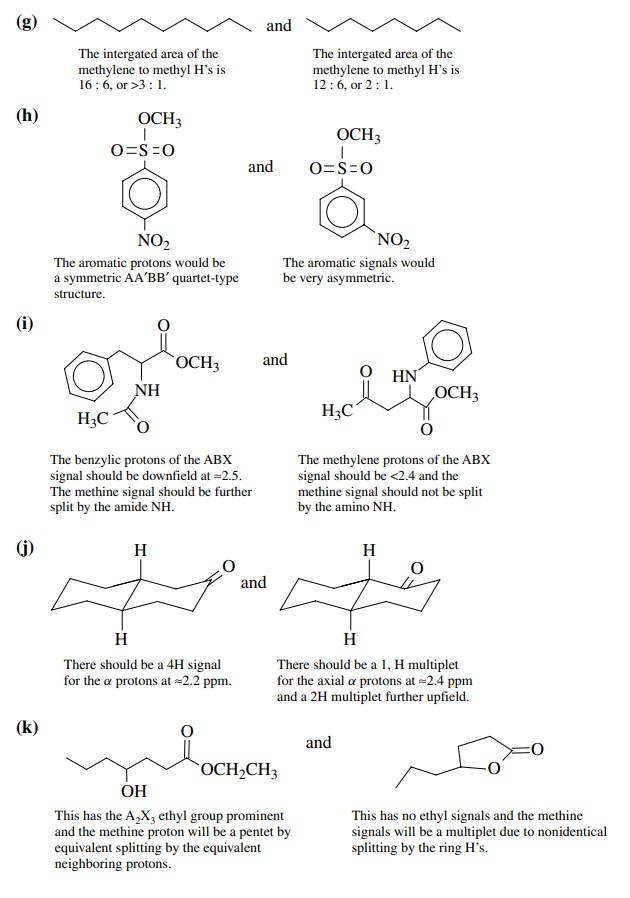11.3. Tell how you could use 13C NMR to distinguish the following pairs of compounds. Be specific as to what data you would look for and how you would interpret it. There might be more than one feature of 13C NMR that could be used so give a complete answer.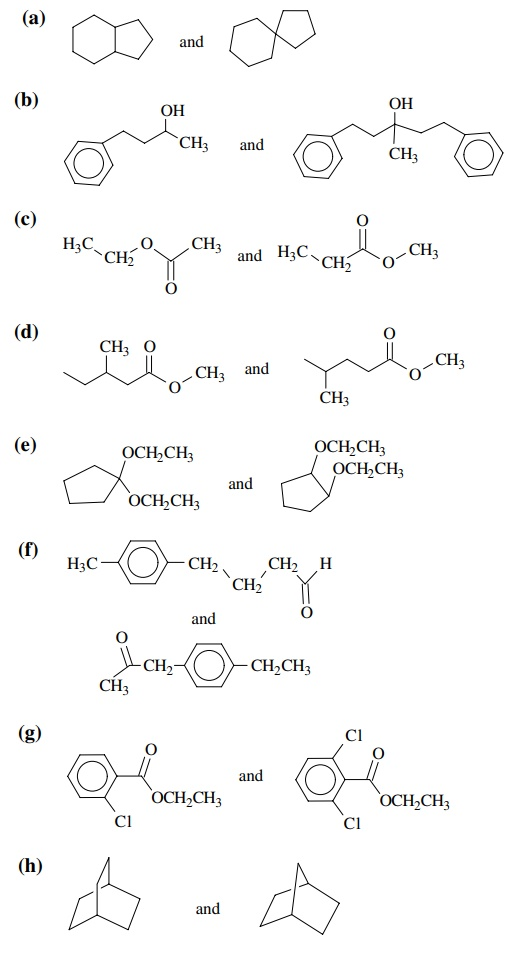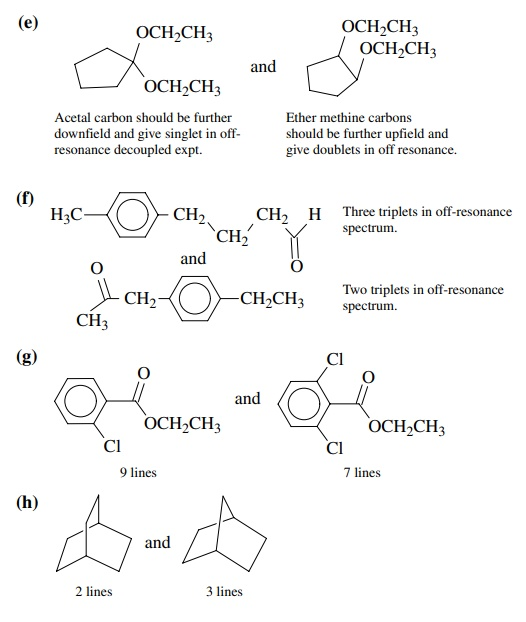11.4. Tell how you could use IR spectroscopy to distinguish the following pairs of compounds. Be specific as to what data you would look for and how you would interpret it. There might be more than one way to distinguish them by IR so give a complete answer.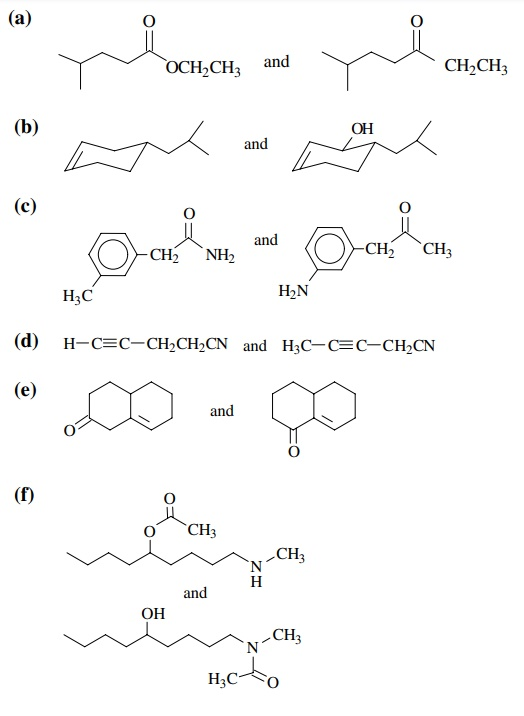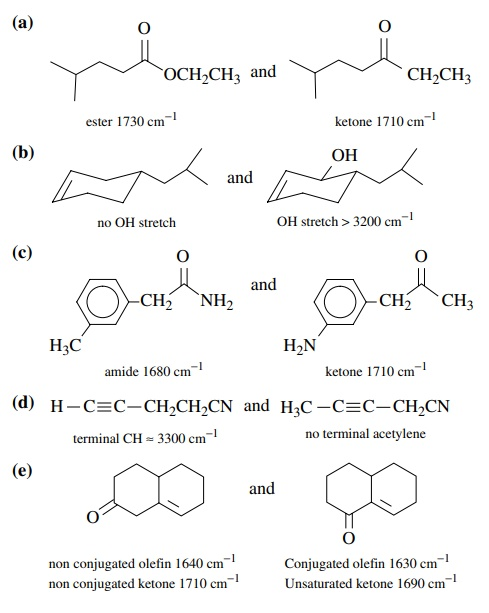11.5. Tell how you could use mass spectrometry (MS) to distinguish the fol-lowing pairs of compounds. Be specific as to what data you would look for and how you would interpret it. There might be more than one way to distinguish them by MS so give a complete answer.11.6. Rationalize the major fragmentation pathways observed for ethyl pheny-lacetate A and diethyl phenylmalonate B.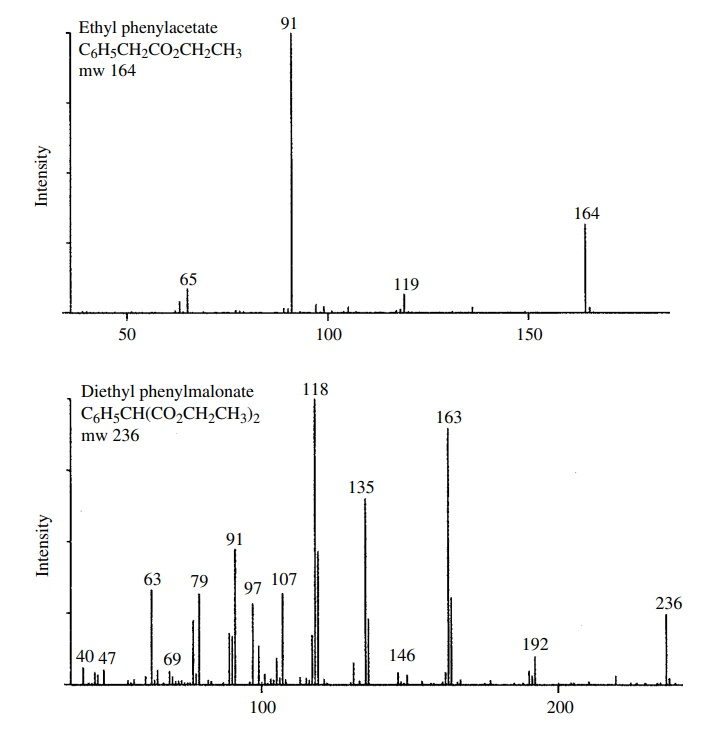11.7. Give two instrumental methods that would permit you to distinguish the following. Explain what data you would use and how it would allow you to make the distinction.